Home > ACC7 > Chapter cc27 > Lesson cc27.1.7 > Problem7-84

7-84.
1. Evaluate the inequality below for the following listed values of x. Decide if the value makes the statement true or false. Show your work. Homework Help ✎

2. 3x − 3 ≥ 2x + 3

1. x = −3

2. x = 9.5

3. x = 6

4. x = 10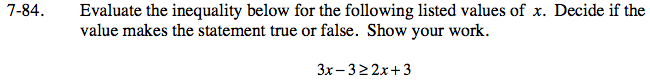Substitute each value in for x and solve the inequality to see if it is true or false.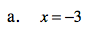3(−3) − 3 ≥ 2(−3) + 3

−9 − 3 ≥ −6 + 3

−12 ≥ −3
False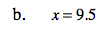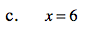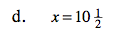True. Be sure to know why this is the answer.